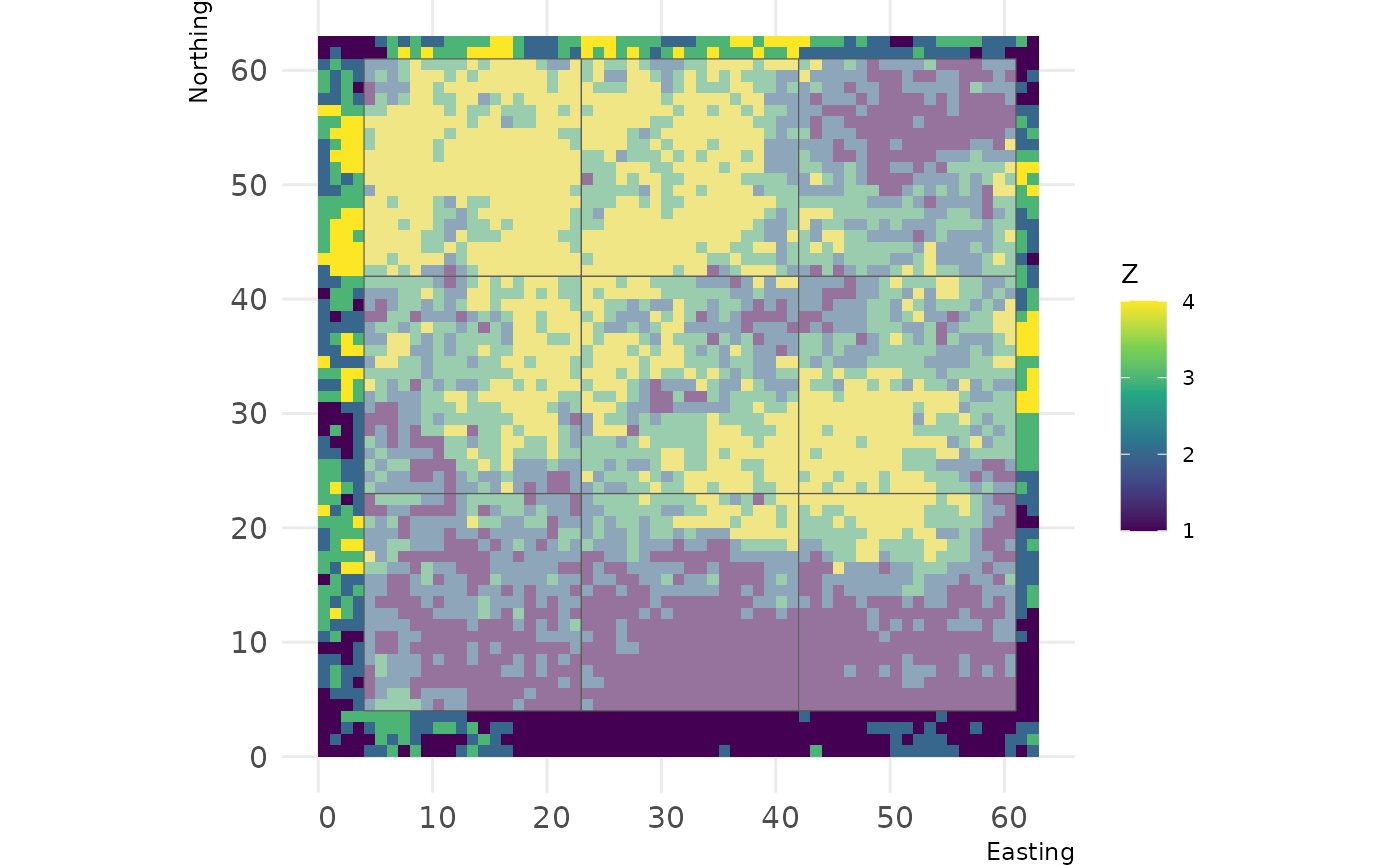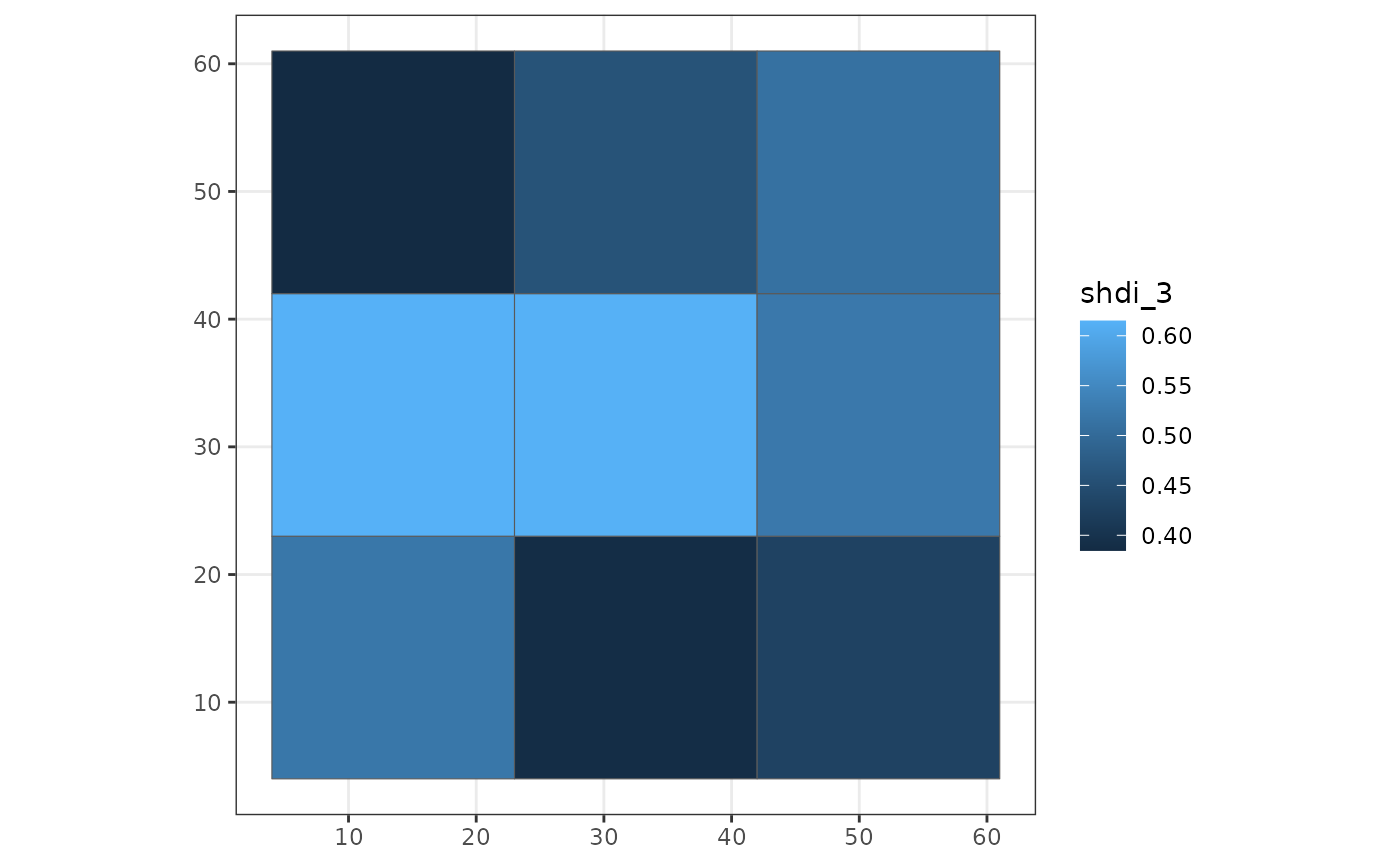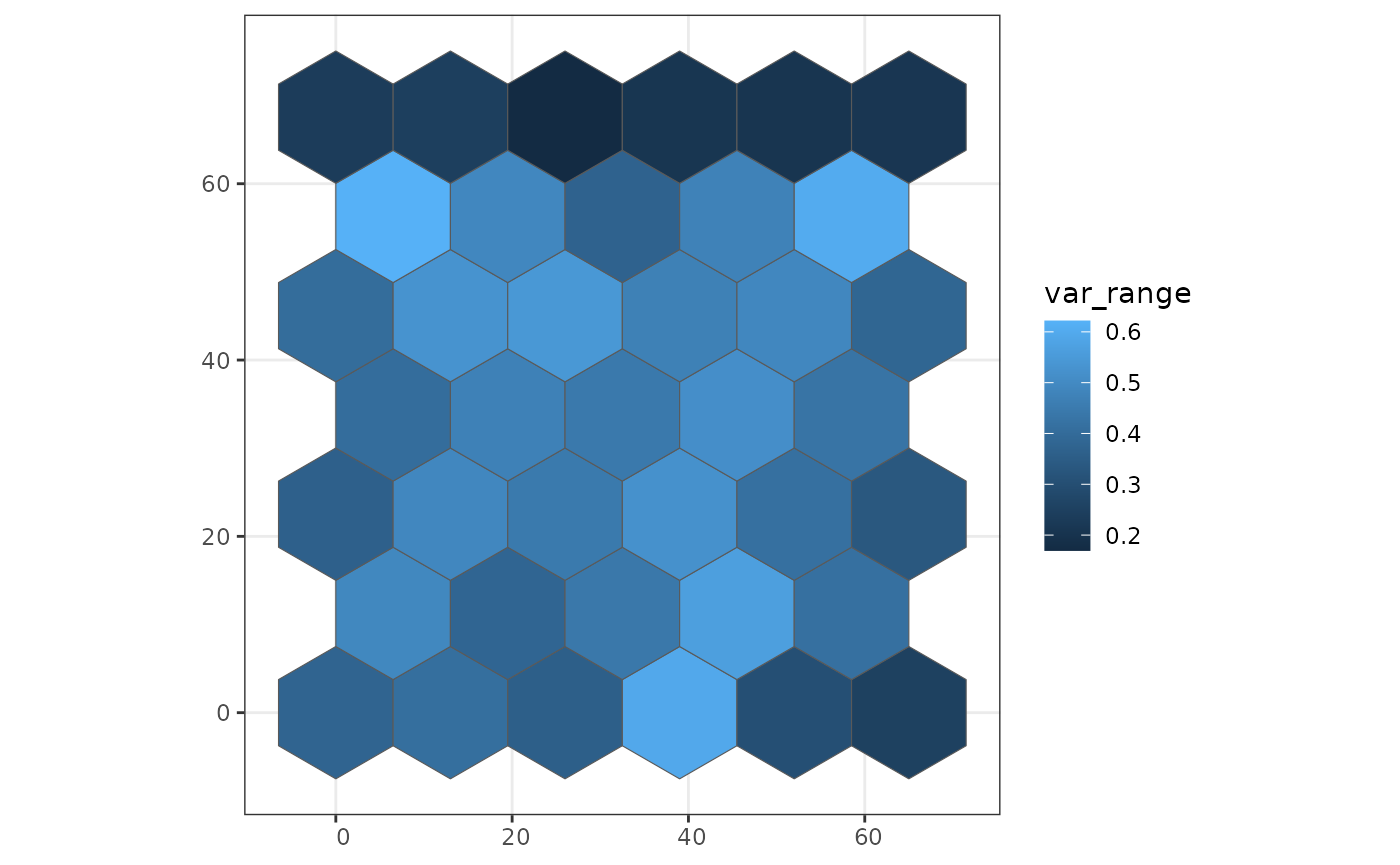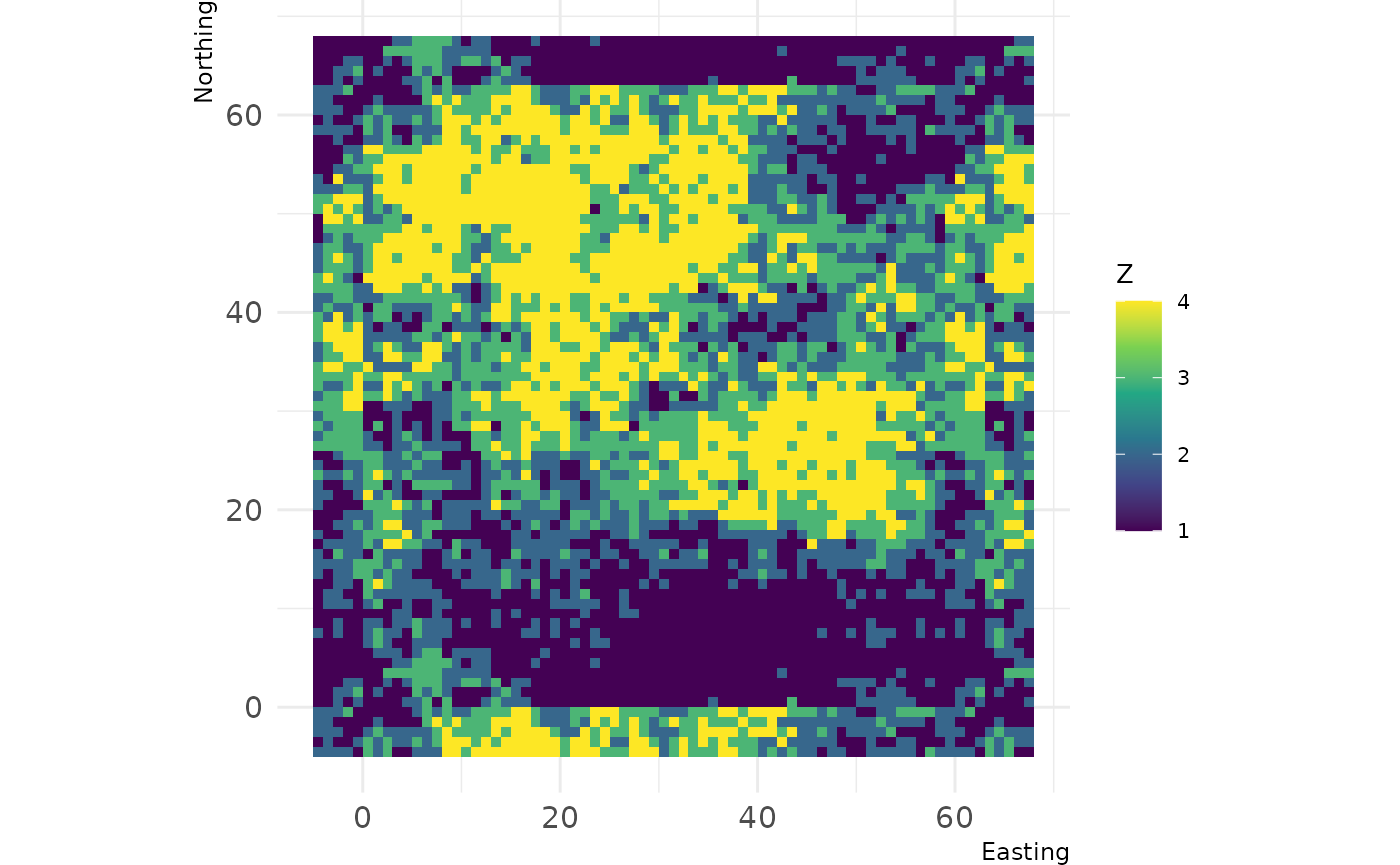## Package overview

The primary functions of the `grainchanger` package are those which facilitate moving-window (`winmove_agg`) and direct (`nomove_agg`) data aggregation. These functions aggregate fine-grain data (`fine_dat`) to a coarse-grain (`coarse_dat`) using a function specified by the user (`agg_fun`). The moving-window method takes in an additional function (`win_fun`) which smooths the fine-grain data prior to aggregation.

The moving-window smoothing function is also available in the package (`winmove`), as well as several built-in functions, and an additional utility function for use with simulated landscapes (`create_torus`).

The `winmove` function acts as a convenient wrapper to `raster::focalWeight` and `raster::focal` which takes advantage of optimised functions built into the `grainchanger` package.

## Data aggregation & `sf`

The functions in `grainchanger` have been written to be compatible with the `sf` package. The online textbook Geocomputation with R provides an introduction to spatial data analysis with the `sf` package.

### Moving-window data aggregation

In this example, we show how to create a coarse grain grid over the fine-grain data using `st_make_grid` from the `sf` package. We then aggregate the package data `cat_ls` to this grid using moving-window data aggregation and plot using `ggplot2`.

``````library(grainchanger)
library(sf)
library(ggplot2)

coarse_dat <- cat_ls %>%
# get the bounding box
st_bbox() %>%
# turn into an sfc object
st_as_sfc() %>%
# negative buffer
st_buffer(-4) %>%
# make a square grid
st_make_grid(cellsize = 19) %>%
# turn into sf object
st_sf()

# we can plot this grid on top of the fine data
landscapetools::show_landscape(cat_ls) +
geom_sf(data = coarse_dat, alpha = 0.5)````````````

coarse_dat\$shdi_3 <- winmove_agg(coarse_dat = coarse_dat,
fine_dat = cat_ls,
d = 3,
type = "rectangle",
win_fun = shdi,
agg_fun = mean,
is_grid = FALSE,
lc_class = 1:4)

ggplot(coarse_dat, aes(fill = shdi_3)) +
geom_sf() +
theme_bw()``````Note that when creating the grid, we made it 4 units smaller (the size of the moving window) than the fine-resolution data. The reason for this is that we avoid edge effects created when the moving window goes beyond the extent of the data. A warning is thrown if `fine_dat` is the same size as (or smaller than) `coarse_dat`. The below is an example of this, using `g_sf` from the package.

``````g_sf\$shei_4 <- winmove_agg(coarse_dat = g_sf,
fine_dat = cat_ls,
d = 4,
type = "rectangle",
win_fun = shei,
agg_fun = mean,
is_grid = FALSE,
lc_class = 1:4)
#> Warning: Moving window extends beyond extent of `fine_dat`
#> You will get edge effects for the following cells of `coarse_dat`:
#> 1,2,3,4,5,6,7,8,9``````

### Direct data aggregation

In this example we show how to read in a shapefile as an `sf` object, apply direct data aggregation, and plot using `ggplot2`. `cont_ls` is a fine-resolution RasterLayer provided with the package.

``````library(sf)
library(ggplot2)

#> Reading layer `poly_sf' from data source
#>   `/usr/lib/R/site-library/grainchanger/shape/poly_sf.shp' using driver `ESRI Shapefile'
#> Simple feature collection with 39 features and 1 field
#> Geometry type: POLYGON
#> Dimension:     XY
#> Bounding box:  xmin: -6.5 ymin: -7.505553 xmax: 71.5 ymax: 75.05553
#> CRS:           NA

coarse_dat\$var_range <- nomove_agg(coarse_dat = coarse_dat,
fine_dat = cont_ls,
agg_fun = var_range,
is_grid = FALSE)

ggplot(coarse_dat, aes(fill = var_range)) +
geom_sf() +
theme_bw()``````## Functions

There are a number of inbuilt functions in the grainchanger package, with their usage outlined below. While it is possible to use user-defined functions within both `winmove_agg` and `nomove_agg`, we welcome suggestions for additional functions. Please add as an issue - doing it this way means we can maximise the speed of the function.

prop Calculate the proportion of a given class lc_class (numeric)
shdi Calculate the Shannon diversity lc_class (numeric)
shei Calculate the Shannon evenness lc_class (numeric)
range Calculate the range of values

### Create torus

The `create_torus` function takes as input a square or rectangular landscape and pads it by a specified radius, creating the effect of a torus. We developed this function in order to avoid edge effects when testing methods on simulated landscapes (such as those from NLMR).

``````torus <- create_torus(cat_ls, 5)

landscapetools::show_landscape(torus)``````### Running aggregation in parallel

The `winmove_agg` and `nomove_agg` functions have been designed to work with the `future` package. This means that with just one additional line of code, the functions can be run in parallel while taking advantage of the resources available to the user.

``````library(future)
plan(multisession)``````

See the `future` documentation for more information.